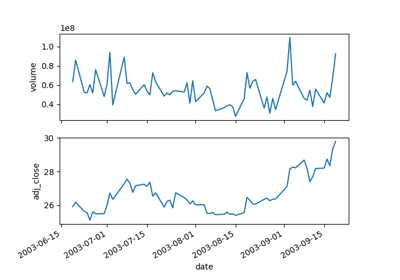You are reading an old version of the documentation (v3.1.0). For the latest version see https://matplotlib.org/stable/

# matplotlib.pyplot.plotfile¶

matplotlib.pyplot.plotfile(fname, cols=(0, ), plotfuncs=None, comments='#', skiprows=0, checkrows=5, delimiter=', ', names=None, subplots=True, newfig=True, **kwargs)[source]

Plot the data in a file.

cols is a sequence of column identifiers to plot. An identifier is either an int or a string. If it is an int, it indicates the column number. If it is a string, it indicates the column header. matplotlib will make column headers lower case, replace spaces with underscores, and remove all illegal characters; so 'Adj Close*' will have name 'adj_close'.

• If len(cols) == 1, only that column will be plotted on the y axis.
• If len(cols) > 1, the first element will be an identifier for data for the x axis and the remaining elements will be the column indexes for multiple subplots if subplots is True (the default), or for lines in a single subplot if subplots is False.

plotfuncs, if not None, is a dictionary mapping identifier to an Axes plotting function as a string. Default is 'plot', other choices are 'semilogy', 'fill', 'bar', etc. You must use the same type of identifier in the cols vector as you use in the plotfuncs dictionary, e.g., integer column numbers in both or column names in both. If subplots is False, then including any function such as 'semilogy' that changes the axis scaling will set the scaling for all columns.

• comments: the character used to indicate the start of a comment in the file, or None to switch off the removal of comments
• skiprows: is the number of rows from the top to skip
• checkrows: is the number of rows to check to validate the column data type. When set to zero all rows are validated.
• delimiter: is the character(s) separating row items
• names: if not None, is a list of header names. In this case, no header will be read from the file

If newfig is True, the plot always will be made in a new figure; if False, it will be made in the current figure if one exists, else in a new figure.

kwargs are passed on to plotting functions.

Example usage:

# plot the 2nd and 4th column against the 1st in two subplots
plotfile(fname, (0,1,3))

# plot using column names; specify an alternate plot type for volume
plotfile(fname, ('date', 'volume', 'adj_close'),
plotfuncs={'volume': 'semilogy'})


Note: plotfile is intended as a convenience for quickly plotting data from flat files; it is not intended as an alternative interface to general plotting with pyplot or matplotlib.

## Examples using matplotlib.pyplot.plotfile¶Plotfile Demo